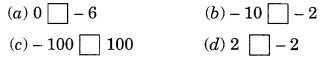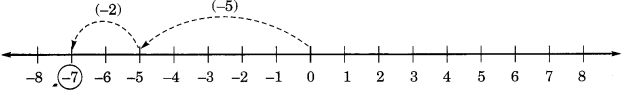# Integers Class 6 Extra Questions Maths Chapter 6

Integers Class 6 Extra Questions Maths Chapter 6

### Integers Class 6 Extra Questions Maths Chapter 6

Extra Questions for Class 6 Maths Chapter 6 Integers

### Integers Class 6 Extra Questions Very Short Answer Type

Question 1.
Represent the following on number line:
(a) -5
(b) 4
Solution:
(a) – 5(b) 4Question 2.
Identify the negative integers from the given numbers.
– 5, 3, 0, 5, – 6, 7, 3, 4, – 4, – 7
Solution:
Negative integers are – 5, – 6, – 4 and – 7 .

Question 3.
What is the additive identity of – 20?
Solution:
Additive identity of – 20 is 20.

Question 4.
What is negative of 0?
Solution:
The negative of 0 is 0 it self.

Question 5.
What is the absolute value of |- 6| ?
Solution:
Absolute value of |- 6| is 6.

Question 6.
What is the absolute value of |0| ?
Solution:
Absolute value of |0| is 0.

Question 7.
What is the negative of – 13?
Solution:
Negative of (- 13) is – (- 13) = 13.

Question 8.
What is the successor of – 7?
Solution:
The successor of -7 = -7 + 1 = -6

Question 9.
What is the predecessor of – 5?
Solution:
The predecessor of -5 is – 5 – 1 = -6.

Question 10.
Write the opposite of 50 km towards north?
Solution:
Opposite of 50 km towards north is 50 km towards south.

### Integers Class 6 Extra Questions Short Answer Type

Question 11.
Write the following integers in their increasing order.
– 3, 0, – 6, 5, – 4, 6, 3, – 8
Solution:
The required increasing order is:
– 8, – 6, – 4, – 3, 0, 3, 5, 6

Question 12.
Comparing the following pairs of number use > or < .Solution:
(a) 0 > – 6
(b) – 10 < – 2
(c) – 100 < 100
(d) 2 > – 2

Question 13.
Write all the integers between the following pair of integers:
(а) 0 and – 4
(B) – 5 and 5
(c) – 8 and – 13
(d) 3 and 6
Solution:
(a) Integers between 0 and – 4 are: -3, -2, -1
(B) Integers between – 5 and 5 are: -4, -3, -2, -1, 0, 1, 2, 3, 4.
(c) Integers between – 8 and – 13 are: -12, -11, -10, -9
(d) Integers between 3 and 6 are: 4 and 5

Question 14.
Find the solution of the following additions using number line:
(a)(- 3) + 5
(B) (- 5) + (-2)
Solution:
(a)(- 3) + 5∴ (-3) + 5 = 2

(B) (- 5) + (-2)∴ (-5) + (-2) = (-7)

Question 15.
Find the sum of the following integers:
(a) (- 8) + (+ 5) + (- 3) + (- 2)
(B) (- 7) + (- 9) + (+ 4) + (+ 3)
Solution:
(a) (- 8) + (+ 5) + (- 3) + (- 2)
= (- 8) + (+ 5) – (3 + 2)
= (- 8) + (+ 5) – (5)
= (- 8) + 0 = – 8 [ ∵ (+ a) + (- a) = 0]

(b) (- 7) + (- 9) + (+ 4) + (+ 3)
= (- 7) + (- 9) + (4 + 3)
= (- 7) + (- 9) + (+ 7)
= (- 7) + (+ 7) + (- 9)
= 0 + (- 9) = – 9 [ ∵ (- a) + (+ a) = 0]

### Integers Class 6 Extra Questions Long Answer Type

Question 16.
Ramesh thinks of an integer. He subtracts 12 from it and gets the result as – 6. What was the integer he thought of?
Solution:
The given sum can be written as under.
(___) – (12) = – 6
The required integer is 12 – 6 = 6.

Question 17.
Fill in the blanks:
(a) To subtract (- 8) from 13, we add ……… to 13.
(b) To subtract 5 from (- 12), we add ……… to (-13)
(c) The negative of a negative integer is a ……… integer.
(d) An integer when added to its opposite gives ……… as the sum.
(e) -4 + …….. = 1
(f) 4 – (- 3) = ………
(g) ……… + (- 79) = 19
Solution:
(a) 8
(b) – 4
(c) positive
(d) 0
(e) 5
(f) 7
(g) 98

Question 18.
Determines:
(a) | 5 | – |- 3 |
(b) | 5 – 6|+ | -1 |
(c) – 7 + | – 3 |
(d) | 5 | + | – 12 |
Solution:
(a) | 5 | – | – 3 | = 5 – 3 = 2 [∵ |a| = a and |- a| = a]
(b) |5 – 6 | + |- 1| = |- 1| + |-1| = 1 + 1 = 2
(c) – 7 + | – 3 | = -7 + 3 = -4
(d) | 5 | + | – 12 | = 5 + 12 = 17

### Integers Class 6 Extra Questions Higher Order Thinking Skills (HOTS)

Question 19.
If * is an operation such that for two integers p and q, p * q = p + q – 2, then find:
(a) 6 * 2
(b) (- 2) * (- 3)
(c) (- 2) * (4)
(d) (+ 3) * (- 1)
Solution:
(a) Given that: p * q = p + q – 2
⇒ 6 * 2 = 6 + 2 – 2 = 6 + 0 = 6
Thus, 6 * 2 = 6.

(b) Given that: p * q = p + q – 2
⇒ (- 2) * (- 3) = (- 2) + (- 3) – 2
= -5 – 2 = -7.
Thus, (- 2) * (- 3) = – 7.

(c) Given that: p * q = p + q – 2
⇒ (- 2) * (4) = (- 2) + (4) – 2 = 2 – 2 = 0.
Thus, (- 2) * (4) = 0.

(d) Given that p*q – p + q – 2
⇒ (+ 3) * (- 1) = (+ 3) + (- 1) – 2 = 2 – 2 = 0
Thus, (+ 3) * (- 1) = 0.

Question 20.
Complete the table:Solution:
The required table can be completed as under:## SabDekho

The Complete Educational Website